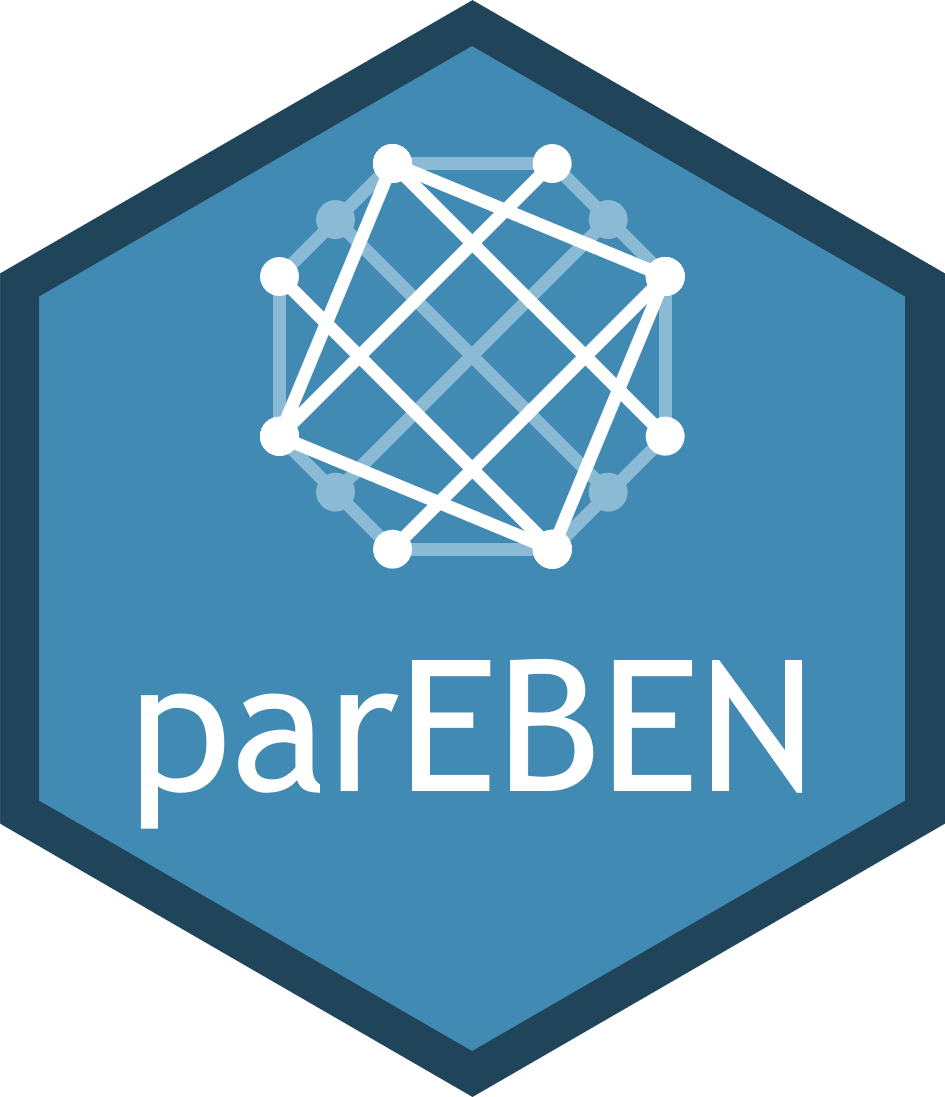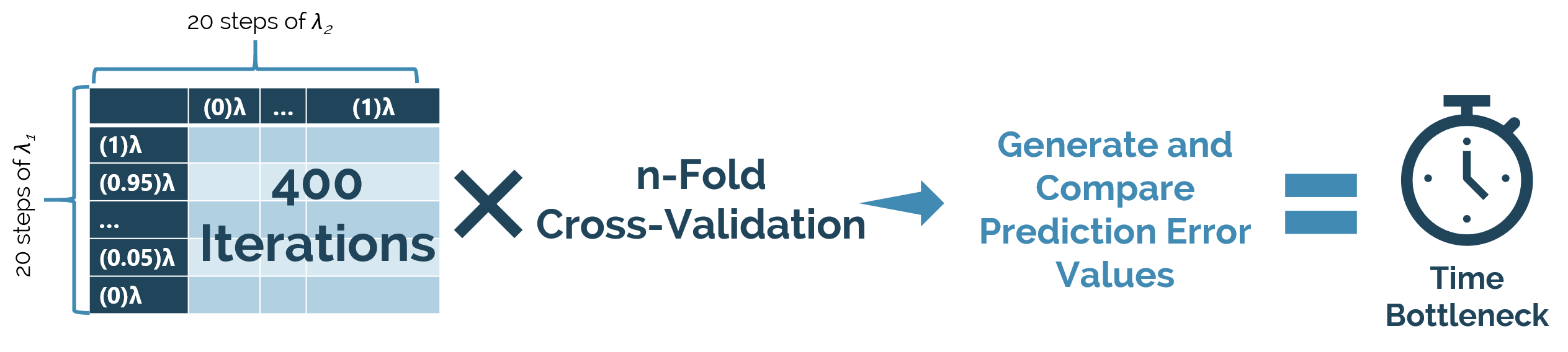{{ message }}

# colbyford / parEBEN

Parallel Implementations of the Empirical Bayesian Elastic Net Cross-Validation in R

## Files

Failed to load latest commit information.
Type
Name
Commit time

# parEBEN - Parallel Implementations of the Empirical Bayesian Elastic Net Cross-Validation in R

### Colby T. Ford, Ph.D.## Abstract

The Empirical Bayesian Elastic Net (EBEN) algorithm was developed by Huang et al. for handling multicollinearity in generalized linear regression models. Historically, this has been used in the analysis of quantitative trait loci (QTLs) and gene-gene interactions (epistasis). In addition to the algorithm, the group also created the EBEN package for R. This package includes functions to generate the elastic nets for both binomial and gaussian priors. These functions are efficient and do not require large amounts of computational time. However, the package also includes functions for the cross-validation of those models. While essential, this step is a considerably more complex task. The cross-validation functions perform a sweep to determine hyperparameters and minimize prediction error. More specifically, an n-fold cross-validation sweep is performed to minimize error by trying combinations of two parameters (α and λ) in a stepped manner. Experimentally, it has been shown that this can take a rather extended amount of time, especially on larger datasets (as seen in genomics problems).To combat this complexity issue, the parallelization of the cross-validation functions was performed by employing parallel packages in R. By parallelizing the iterations of the cross-validation over multiple CPU cores or multiple machines of a computing clusters, a drastic time reduction can seen with no negative effect on the resulting EBEN models. By reducing the computation time, regression models on larger, more complex data can be completed without such a delay. This also opens the door for larger datasets to be analyzed as opposed to limiting the research due to time and computing resource constraints. Thus, parallelizing the cross-validation of the EBEN models will prove to be greatly beneficial in future research using cross-validated Bayesian elastic nets.

## Time Reduction Benchmark

To interactively view cross-validation time benchmarks between parEBEN and the original EBEN package, click here

## Installation

You can install the latest stable version from GitHub using the following command:

```library(devtools)
install_github("colbyford/parEBEN")
library(parEBEN)```

## Usage

First, select the parallelization method you wish to use. Currently, all foreach-related methods are supported such as doParallel, doMPI, and doSNOW.

### Initialize The Cluster

Note: Refer to the manual for your desired foreach parallelization package as the initialization may differ between methods.

##### Local Parallel
```library(doParallel)
no_cores <- detectCores()
cl <- makeCluster(no_cores)
#clusterExport(cl, c("CrossValidate"))
registerDoParallel(cl)```
##### Cluster Distribution
```library(doMPI)
# create and register a doMPI cluster if necessary
if (!identical(getDoParName(), 'doMPI')) {
# set count to (cores_requested-1)
cl <- startMPIcluster(count=255,verbose=TRUE)
registerDoMPI(cl)
}```
##### Microsoft Machine Learning Server Distribution
``````## Set your compute contaxt as Spark, local parallel, MapReduce, etc.
### See: https://docs.microsoft.com/en-us/machine-learning-server/r-reference/revoscaler/rxspark
### Sample Code: https://gist.github.com/premalxyz/e97ae7823052b7a426cb816830c0188c#file-spark_compute_context-r

mySparkCluster <- RxSpark(ClusterInfo)
rxSetComputeContext(mySparkCluster)

## Register the context using doRSR
library(doRSR)
registerDoRSR()
``````

### Begin the Cross-Validation

```## Load in data and required EBEN and parEBEN packages
library(EBEN)
library(parEBEN)

## Create small sample matrix for testing
data(BASIS)
data(y)
n = 50
k = 100
BASIS = BASIS[1:n,1:k]
y  = y[1:n]

parEBENcv <- CrossValidate(BASIS,
y,
nFolds = 3,
Epis = "no",
prior = "gaussian",
search = "global"
)

## Use the optimal values in the EBEN model
EBENoutput <- EBelasticNet.Gaussian(BASIS,
y,
lambda = parEBENcv\$lambda.optimal,
alpha = parEBENcv\$alpha.optimal,
Epis = "no",
verbose = 1)```

## To Do List

• Binomial prior cross-validation script with doParallel.
• Gaussian prior cross-validation script with doParallel.
• Binomial prior cross-validation script with doMPI.
• Gaussian prior cross-validation script with doMPI.
• Binomial prior cross-validation script with Microsoft ML Server (RevoScaleR/doRSR).
• Gaussian prior cross-validation script with Microsoft ML Server (RevoScaleR/doRSR).
• Binomial prior cross-validation script with SparkR.
• Gaussian prior cross-validation script with SparkR.
• Binomial prior cross-validation script with CUDA.
• Gaussian prior cross-validation script with CUDA.
• Manual File/Usage Instructions.

## Publication and How To Cite

Data and materials used in publication can be found here.

``````Jia Wen, Colby T Ford, Daniel Janies, Xinghua Shi, A Parallelized Strategy for Epistasis Analysis Based on Empirical Bayesian Elastic Net Models, Bioinformatics, , btaa216, https://doi.org/10.1093/bioinformatics/btaa216
``````

or using BibTeX...

``````@article{10.1093/bioinformatics/btaa216,
author = {Wen, Jia and Ford, Colby T and Janies, Daniel and Shi, Xinghua},
title = "{A Parallelized Strategy for Epistasis Analysis Based on Empirical Bayesian Elastic Net Models}",
journal = {Bioinformatics},
year = {2020},
month = {03},
issn = {1367-4803},
doi = {10.1093/bioinformatics/btaa216},
url = {https://doi.org/10.1093/bioinformatics/btaa216},
note = {btaa216},
}
``````

## Funding

This project was funded in part by NIH R15HG009565.

Parallel Implementations of the Empirical Bayesian Elastic Net Cross-Validation in R